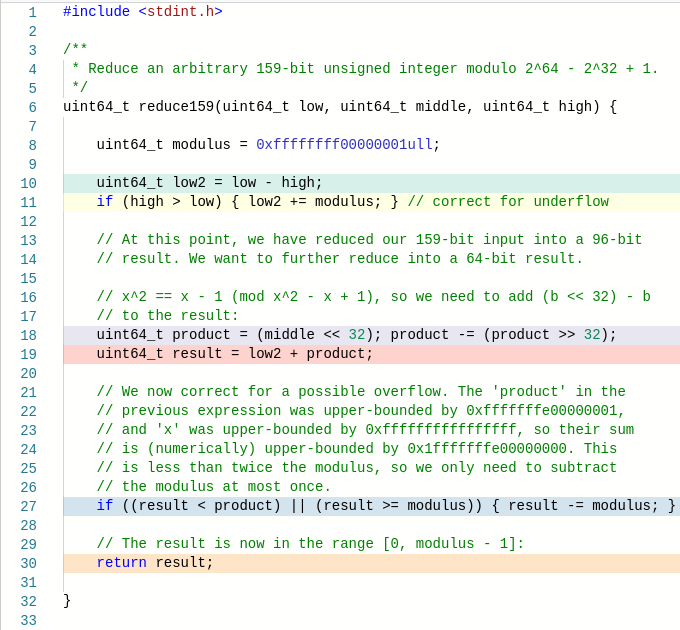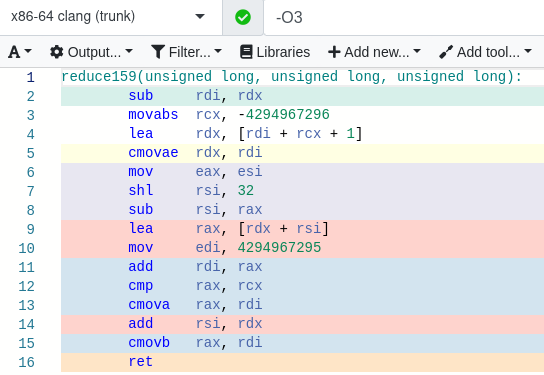# An efficient prime for number-theoretic transforms

My new favourite prime is 18446744069414584321.

It is given by$p = \Phi_6(x) = x^2 - x + 1$, where$x = 2^{32}$. This means that, in the finite field$\mathbb{F}_p$, 2^32 functions as a primitive 6th root of unity, and therefore 2 is a primitive 192nd root of unity. It turns out that this field possesses multiple properties which make it well-suited to performing number-theoretic transforms (the finite field analogue of the Fast Fourier Transform).

### Arithmetic

Firstly, note that arithmetic is especially convenient in this field. An element of the field can be represented as an unsigned 64-bit integer, since p is slightly less than 2^64. We can also efficiently reduce modulo p without involving multiplication or division. In particular, if we have a non-negative integer n less than 2^159, then we can write it in the form:$n = A 2^{96} + B 2^{64} + C$

where A is a 63-bit integer, B is a 32-bit integer, and C is a 64-bit integer. Since 2^96 is congruent to −1 modulo p, we can then rewrite this as:$B 2^{64} + (C - A)$

If A happened to be larger than C, and therefore the result of the subtraction underflowed, then we can correct for this by adding p to the result. Now we have a 96-bit integer, and wish to reduce it further to a 64-bit integer less than p. To do this, we note that 2^64 is congruent to 2^32 − 1, so we can multiply B by 2^32 using a binary shift and a subtraction, and then add it to the result. We might encounter an overflow, but we can correct for that by subtracting p.

In C/C++, the algorithm is as follows:This involves no multiplication or division instructions. Indeed, we can take a look at the compiled assembly code by using the Godbolt Compiler Explorer:Observe that, using LLVM as the compiler, the resulting code is branchless (despite the word ‘if’ appearing twice in the source code) and all of the instructions are particularly cheap.

Now, why would we end up with a 159-bit integer in the first place and want to reduce it? There are two occasions where this subroutine is useful:

• To perform a multiplication modulo p, we use machine instructions to multiply the two 64-bit operands to give a 128-bit result.
• Left-shifting a 64-bit integer by up to 95 bits gives a 159-bit result.

The latter allows us to cheaply multiply an element of our field by any power of 2. In particular, if we want to multiply by 2^(96a + b), then do the following:

• if a is odd, then subtract the input from p (in-place), relying on the fact that 2^96 is congruent to −1;
• shift-left by b bits (to give a 159-bit result) and reduce using the subroutine.

That is to say, multiplying by any 192nd root of unity is ‘fast’, bypassing the need to perform a general multiplication. (This is particularly useful for GPUs, where there isn’t a 64-bit multiplier in hardware, so multiplication expands to many invocations of the hardware multiplier.)

### Other roots of unity

As well as possessing these 192nd roots of unity, the field also contains roots of unity of any order dividing p − 1. This factorises as follows:$p - 1 = 2^{32} \times 3 \times 5 \times 17 \times 257 \times 65537$

where those odd prime factors are the five known Fermat primes. We can perform a Fourier transform of any length that divides p − 1, since all of the requisite roots of unity exist, but this will be especially efficient if we only involve the smaller prime factors.

Most of these other roots of unity are ‘slow’, meaning that there is no obvious way to multiply by them without performing a general multiplication. There is, however, a nice observation by Schönhage:$\sqrt{2} = \zeta + \zeta^7$, where ζ is a primitive 8th root of unity, so we can efficiently multiply by the square-root of 2 (which is, of course, a primitive 384th root of unity).

A length-N FFT involves the Nth roots of unity, so we would want to use FFTs of length dividing 192 (or 384 using the Schönhage trick) whenever possible. This suggests the following algorithm for convolving two sequences:

• choose an FFT length N that divides$3 \times 2^{32}$ and is large enough to accommodate the result of the convolution;
• write N as a product of (at most 5) numbers dividing 384. For example, in the largest case we could write N = 128 × 128 × 128 × 96 × 64;
• use this decomposition as the basis of a mixed-radix Cooley-Tukey FFT. That is to say, we apply:
• N/128 FFTs of length 128;
• N/128 FFTs of length 128;
• N/128 FFTs of length 128;
• N/96 FFTs of length 96;
• N/64 FFTs of length 64.

Since there are at most 5 rounds of FFTs, we only need to apply arbitrary twiddle-factors (which can be precomputed and stored in a table) 4 times, i.e. between successive rounds of FFTs. Compare this to an FFT over the complex numbers, where there are only 4 ‘fast’ roots of unity (±1 and ±i) and therefore irrational twiddle factors must be applied much more often.

### Fürer’s algorithm

Martin Fürer’s fast integer multiplication algorithm uses this idea recursively, expressing the integer multiplication problem as a convolution over a suitably chosen ring with plenty of fast roots of unity. In particular, he writes:

We will achieve the desired speed-up by working over a ring with many “easy” powers of ω. Hence, the new faster integer multiplication algorithm is based on two key ideas:

• An FFT version is used with the property that most occurring roots of unity are of low order.

• The computation is done over a ring where multiplications with many low order roots of unity are very simple and can be implemented as a kind of cyclic shifts. At the same time, this ring also contains high order roots of unity.

The field$\mathbb{F}_p$ for$p = 2^{64} - 2^{32} + 1$ is a fixed-size ring with these desirable properties. It supports multiplication of integers up to$3 \times 2^{35}$ bits (12 GB) by writing them in ‘balanced base 2^16’ (where each ‘digit’ can be between −32768 and 32767). Each of the two integers will occupy at most$3 \times 2^{31}$ digits, so the result can be computed by a convolution of length$3 \times 2^{32}$. The maximum absolute value of a coefficient that could occur as a result of such a convolution is$3 \times 2^{61} < \frac{1}{2} p$, so the coefficient can be recovered exactly if the convolution is performed over$\mathbb{F}_p$ by reducing each coefficient into the range [−p/2, +p/2].

Of course, Fürer’s paper was interested in the asymptotics of integer multiplication, so it needs to work for arbitrarily large integers (not just integers of size at most 12 GB). The remaining idea was therefore to apply this idea recursively: to split a length-N integer into chunks of size O(log N), and use Fürer’s algorithm itself to handle those chunks (by splitting into chunks of size O(log log N), and so forth, until reaching a ‘base case’ where the integers are sufficiently small that FFT-based methods are unhelpful).

The number of layers of recursion is therefore the number of times you need to iteratively take the logarithm of N before log log log … log log N < K, where K is the base-case cutoff point. This is called the iterated logarithm, and denoted$\log^{\star} N$.

Each layer of recursion in Fürer’s algorithm costs a constant factor F more than the previous layer, so the overall complexity is$O(N \log N F^{\log^{\star} N})$. It was the asymptotically fastest multiplication algorithm between its invention in 2007 (taking the crown from Schönhage-Strassen) and 2016 (when an O(N log N) algorithm was discovered).

### Practical integer multiplication

In practice, large integer multiplication tends to use either Schönhage-Strassen (in the case of the GNU Multiprecision Library), or floating-point FFTs, or a bunch of number-theoretic transforms over different primes with a final application of the Chinese Remainder Theorem (in the case of Alexander Yee’s y-cruncher). The primes used by these number-theoretic transforms don’t support any ‘fast’ roots of unity, though; all of the twiddle factors are applied using multiplications.

This suggests that a number-theoretic transform over the field of 18446744069414584321 elements may indeed be competitive for the sizes of integers it supports (e.g. up to 12 GB). For larger integers, we can use the Schönhage-Strassen algorithm with this prime field as a base case (one layer of Schönhage-Strassen being more than enough to support multiplication of integers that can be stored in practice).

This entry was posted in Uncategorized. Bookmark the permalink.

### 8 Responses to An efficient prime for number-theoretic transforms

1.Catisfluffy says:

Link to code in Godbolt: https://godbolt.org/z/fPKs7nTK6

2.Dan Klishch says:

Is there an implementation of this NTT which is faster than SSA? I was trying very hard to optimize the described algorithm but was only able to get version 5/3 times slower than GMP’s SSA. (See SerenityOS PR #16126 on GitHub)

3.pzq_alex says:

The radix-64 NTT only requires at most four rounds of twiddle factors; however for scalar architectures one may want to use a smaller radix, e.g. 4. However, when using a radix of 4, much more rounds of twiddle factors need to be multiplied. Is there a way to use a smaller radix while retaining the small number of twiddle factors?

•Dan Klishch says:

There is no need to use “difficult” twiddle factors in between of all the NTTs. If implemented correctly, all but original 4 twiddle factors multiplication passes will use powers of 2.
(My implementation does exactly that, although it won’t be particularly useful as a reference)

4.pzq_alex says:

5.spoon says:
•pzq_alex says: